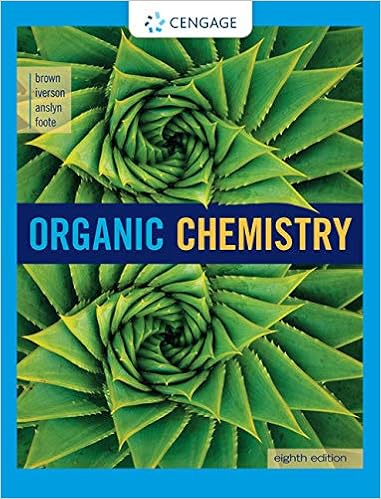Practice Prelim #2 - CHEM 1007 Optional practice problems...

• Test Prep
• 10
• 100% (2) 2 out of 2 people found this document helpful

This preview shows page 1 - 2 out of 10 pages.

We have textbook solutions for you!
The document you are viewing contains questions related to this textbook.The document you are viewing contains questions related to this textbook.
Chapter 5 / Exercise 5.12
Organic Chemistry
BrownExpert Verified
CHEM 1007: Optional practice problems for the second chem 2070 prelim exam The following questions come from several sources. Most come from other general chemistry textbooks. None of these questions were written by Professors Zax or Abruna. These questions are not meant to cover every topic that may be on the prelim, but hopefully these questions will provide some review and problem-solving practice. 1. The first electron affinity of bromine (Br) releases 324.6 kJ/mole ( H = -324.6 kJ/mole ). The production of gaseous bromine ions from bromine molecules can be considered a two-step process in which the first step involves dissociating Br 2 (g) to make 2Br(g), requiring 193 kJ/mole. Br 2 (g) 2Br(g) H = +193 kJ/mole Does the formation of Br - (g) from Br 2 (g) release energy (exothermic) or require added energy (endothermic)? How much energy is added or released in the process of producing one mole of Br - (g)? 2. Given the table of electron affinities and ionization energies below Element First ionization energy First electron affinity Ion Radius Chlorine (Cl) 2.08 aJ -0.580 aJ 133 pm Sodium (Na) 0.83 aJ 0.088 aJ 102 pm (a) Calculate the energy released in the following process: Na(g) + Cl(g) Na + (g) + Cl - (g) (b) Calculate the energy released in the following process: Na(g) + Cl(g) Na + Cl - (g) 3. Using the table of values in problem #2. When sodium chloride (NaCl) is heated in a flame, the flame takes on a yellow color. Calculate the energy associated with this reaction, shown below. The reaction that occurs in the gaseous state is: Na + (g) + Cl - (g) Na(g) + Cl(g) Find the wavelength of light that emits this energy in one photon. 4. Write Lewis structures that show shape for the following molecules. Give the approximate bond angle in each molecule and state whether or not the molecule is polar. (a) SiF 4 (b) XeF 5 (C) SOCl 2 (d) TeF 4 (e) NO 3 - (f) SCl 2 5. In the XF 5 - structure below, element “X” is in the 5 th period. Identify element “X” based on the number of valence electrons in the ion. Then redraw the Lewis structure of the molecule (replacing X with the actual atom symbol) using lines, wedges, and dashes to indicate the molecular shape. Give the name of the molecule’s molecular shape. Give the approximate bond angles in the molecule around the center atom. +
We have textbook solutions for you!
The document you are viewing contains questions related to this textbook.The document you are viewing contains questions related to this textbook.
Chapter 5 / Exercise 5.12
Organic Chemistry
BrownExpert Verified
•••